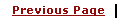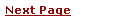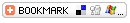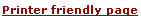C - Pointing to data, Pointer Concept, Learning Pointer, Pointer TutorialLearning C
C Function References
C Useful Resources# C - Pointing to DataA pointer is a special kind of variable. Pointers are designed for storing memory address i.e. the address of another variable. Declaring a pointer is the same as declaring a normal variable except you stick an asterisk '*' in front of the variables identifier.

• There are two new operators you will need to know to work with pointers. The "address of" operator '&' and the "dereferencing" operator '*'. Both are prefix unary operators.

• When you place an ampersand in front of a variable you will get it's address, this can be stored in a pointer vairable.

• When you place an asterisk in front of a pointer you will get the value at the memory address pointed to.

Here is an example to understand what I have stated above.

 ```#include int main() { int my_variable = 6, other_variable = 10; int *my_pointer; printf("the address of my_variable is : %p\n", &my_variable); printf("the address of other_variable is : %p\n", &other_variable); my_pointer = &my_variable; printf("\nafter \"my_pointer = &my_variable\":\n"); printf("\tthe value of my_pointer is %p\n", my_pointer); printf("\tthe value at that address is %d\n", *my_pointer); my_pointer = &other_variable; printf("\nafter \"my_pointer = &other_variable\":\n"); printf("\tthe value of my_pointer is %p\n", my_pointer); printf("\tthe value at that address is %d\n", *my_pointer); return 0; } ```

This will produce following result.

 ```the address of my_variable is : 0xbfffdac4 the address of other_variable is : 0xbfffdac0 after "my_pointer = &my_variable": the value of my_pointer is 0xbfffdac4 the value at that address is 6 after "my_pointer = &other_variable": the value of my_pointer is 0xbfffdac0 the value at that address is 10 ```

# Pointers and Arrays

The most frequent use of pointers in C is for walking efficiently along arrays. In fact, in the implementation of an array, the array name represents the address of the zeroth element of the array, so you can't use it on the left side of an expression. For example:

 ``` char *y; char x; ```

y is of type pointer to character (although it doesn't yet point anywhere). We can make y point to an element of x by either of

 ``` y = &x; y = x; ```

Since x is the address of x this is legal and consistent. Now `*y' gives x. More importantly notice the following:

 ``` *(y+1) gives x *(y+i) gives x[i] and the sequence y = &x; y++; leaves y pointing at x. ```

# Pointer Arithmetic:

C is one of the few languages that allows pointer arithmetic. In other words, you actually move the pointer reference by an arithmetic operation. For example:

 ``` int x = 5, *ip = &x; ip++; ```

On a typical 32-bit machine, *ip would be pointing to 5 after initialization. But ip++; increments the pointer 32-bits or 4-bytes. So whatever was in the next 4-bytes, *ip would be pointing at it.

Pointer arithmetic is very useful when dealing with arrays, because arrays and pointers share a special relationship in C.

# Using Pointer Arithmetic With Arrays:

Arrays occupy consecutive memory slots in the computer's memory. This is where pointer arithmetic comes in handy - if you create a pointer to the first element, incrementing it one step will make it point to the next element.

 ```#include int main() { int *ptr; int arrayInts = {1,2,3,4,5,6,7,8,9,10}; ptr = arrayInts; /* ptr = &arrayInts; is also fine */ printf("The pointer is pointing to the first "); printf("array element, which is %d.\n", *ptr); printf("Let's increment it.....\n"); ptr++; printf("Now it should point to the next element,"); printf(" which is %d.\n", *ptr); printf("But suppose we point to the 3rd and 4th: %d %d.\n", *(ptr+1),*(ptr+2)); ptr+=2; printf("Now skip the next 4 to point to the 8th: %d.\n", *(ptr+=4)); ptr--; printf("Did I miss out my lucky number %d?!\n", *(ptr++)); printf("Back to the 8th it is then..... %d.\n", *ptr); return 0; } ```

This will produce following result:

 ```The pointer is pointing to the first array element, which is 1. Let's increment it..... Now it should point to the next element, which is 2. But suppose we point to the 3rd and 4th: 3 4. Now skip the next 4 to point to the 8th: 8. Did I miss out my lucky number 7?! Back to the 8th it is then..... 8. ```

See more examples on Pointers and Array

# Pointers and const Type Qualifier:

• The const type qualifier can make things a little confusing when it is used with pointer declarations.
• The below example:
 ``` const int * const ip; /* The pointer *ip is const and it points at is also cont */ int * const ip; /* The pointer *ip is const */ const int * ip; /* What *ip is pointing at is const */ int * ip; /* Nothing is const */ ```

As you can see, you must be careful when specifying the const qualifier when using pointers.

# Modifying Variables Using Pointers:

You know how to access the value pointed to using the dereference operator, but you can also modify the content of variables. To achieve this, put the dereferenced pointer on the left of the assignment operator, as shown in this example, which uses an array:

 ```#include int main() { char *ptr; char arrayChars = {'F','r','i','e','n','d','s','\0'}; ptr = arrayChars; printf("The array reads %s.\n", arrayChars); printf("Let's change it..... "); *ptr = 'f'; /* ptr points to the first element */ printf(" now it reads %s.\n", arrayChars); printf("The 3rd character of the array is %c.\n", *(ptr+=2)); printf("Let's change it again..... "); *(ptr - 1) = ' '; printf("Now it reads %s.\n", arrayChars); return 0; } ```

This will produce following result:

 ```The array reads Friends. Let's change it..... now it reads friends. The 3rd character of the array is i. Let's change it again..... Now it reads f iends. ```

# Generic Pointers: ( void Pointer )

When a variable is declared as being a pointer to type void it is known as a generic pointer. Since you cannot have a variable of type void, the pointer will not point to any data and therefore cannot be dereferenced. It is still a pointer though, to use it you just have to cast it to another kind of pointer first. Hence the term Generic pointer. This is very useful when you want a pointer to point to data of different types at different times.

Try the following code to understand Generic Pointers.

 ```#include int main() { int i; char c; void *the_data; i = 6; c = 'a'; the_data = &i; printf("the_data points to the integer value %d\n", *(int*) the_data); the_data = &c; printf("the_data now points to the character %c\n", *(char*) the_data); return 0; } ```

NOTE-1 : Here in first print statement, the_data is prefixed by *(int*). This is called type casting in C language.Type is used to caste a variable from one data type to another datatype to make it compatible to the lvalue.

NOTE-2 : lvalue is something which is used to left side of a statement and in which we can assign some value. A constant can't be an lvalue because we can not assign any value in contact. For example x = y, here x is lvalue and y is rvalue.

However, above example will produce following result:

 ```the_data points to the integer value 6 the_data now points to the character a ```